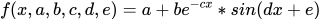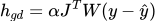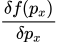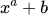# Nonlinear least squares curve fitting in Julia

One commenter on my arduino curve-fitting post asked if it was possible to fit to nonlinear functions as well, such as a decaying sine wave. I said I had no idea, so I did some research and found the Levenberg-Marquardt algorithm.

The LM algorithm takes an n-parameter mathematical function, m observed data points and a guess at the function’s n parameters and updates the parameters at each iteration to get the function output points as close to the observed data points as possible.

For example, the decaying sine wave is given byThese parameters a, b, c, d and e must be started with some guess, which is hopefully close to the actual values. Since the algorithm is a gradient descent at its heart, sometimes it will not “converge”, meaning the results get further away from the best answer and can blow up into huge numbers. As a result it’s better to put good guesses in for the initial parameters rather than just ones.

This is a combination algorithm that changes between using Gradient Descent for slow approach to the solution with bad early guesses, and the Gauss-Newton method for fast acceleration once it’s close to the solution.

Before I could implement this algorithm in C for memory-constrained microcontrollers. I thought I should probably implement it in a “productive” language first. I’ve been meaning to learn Julia, so this was the perfect opportunity. Julia is a language for scientific computing, it’s fast and dynamic.

## Paper to Notebook

One feature I love from Julia, apart from its speed, is its support for Jupyter notebooks. This is the closest we can get to a REPL interface, minimising the development feedback loop. Making the time taken from reading the paper to implementing the algorithm in a notebook so much faster. Let’s dive in to implementing this algorithm!

This algorithm one that’s run as long as we want, or until we reach convergence. Convergence is where we’ve got close enough to the correct result by some chosen measure.

The paper we’ll follow is here, it starts by defining the cost function for the nonlinear least squares. We’ll skip over this bit, we’re interested in the gradient descent method first, which in the paper is derived and then simplified towhere h is the n-length parameter update vector that’s added to our parameters each step.

On the other side of the equation, alpha is just a constant factor that affects how big our steps are taken down the gradient. This is also known as the learning rate in other applications.

W is the nxn weight matrix given to each parameter, in this case and most we will leave it as the identity matrix, which basically means it has no effect, and weights each parameter equally. Y is the observed m datapoints at x that we’re fitting the data to, and ŷ is the model dataponts (from the function).

The function in this case is the decaying sine wave as above, I’ve written it in Julia to deal with a vector of x values and not just one, to make the rest of the math easy:

``f(x, a, b, c, d, e) = a.+b.*exp.(-c.*x) .* sin.(d.*x.+e)``

All those dots next to each operator mean “do this operation on each value in the array”. This means we can pass an array of x-values to the function and receive an array of y-values.

I’ve left the big one til last, J is the Jacobian matrix. It’s quite easy to get confused with this one, I certainly did! The Jacobian matrix is nxm (number of parameters by number of datapoints) in size, and each value is the partial derivative of the function f at each row’s x value, and changing each column’s parameter value.

Enough talking, let’s jump into the code!

First of all to calculate the Jacobian Matrix J each iteration, we need to perturb each parameter by a very small set amount, which we can use to approximate the derivative at each point. This is called the Finite Difference Method.

``````function perturbparameters(parameters, perturbation)
len = length(parameters)
perturbedmatrix = Array{Float64}(undef, len, len)
for i=1:len
for j=1:len
perturbedmatrix[i, j] = parameters[j]
if i == j
perturbedmatrix[i, j] += perturbation
end
end
end
return perturbedmatrix
end``````

`perturbparameters` is a helper function that will take a vector of parameters, a perturbation, and give use back a square matrix of parameters back, where in each row one of the parameters has been perturbed.

For example

`perturbparameters([1 2 3], 0.001)`

returnsSo each row can be used to calculate the partial derivative of the parameter it perturbs. Simple!

Now to calculate the Jacobian, for each x value we need to calculate the partial derivative with respect to each parameterwith a bit of matrix multiplication, we can simplify this with the perturbed parameter matrix and our `f` function that takes a vector of x-values

``````function jacobian(f, x, parameters, perturbation=0.00001)
J = Array{Float64}(undef, length(x), length(parameters))
perturbedparams = perturbparameters(parameters, perturbation)
for i=1:length(parameters)
J[:, i] =  (f(x, perturbedparams[i, :]...) - f(x, parameters...)) / perturbation
end
return J
end``````

Here we pre-allocate the J matrix, since we know its size beforehand. We get the matrix of perturbed parameters, and for each parameter we take the y-values for the perturbed parameters and take the current y values away, dividing by the perturbation. This single line calculates a whole column for our Jacobian Matrix.

If we take a simple exampleWe would expect the Jacobian to show that the derivative of `a` is nonlinear, and the derivative of `b` to be linear, and it is!

``jacobian(squared, 1:5, p, perturbation)``

returns

``````5×2 Array{Float64,2}:
0.0      1.0
1.38634  1.0
3.29602  1.0
5.54556  1.0
8.04784  1.0``````

This is probably enough for one blog post, but the whole algorithm implementation is here, and you can play around with the code on the binder repo here.

If you’re interested in more algorithm implementation, let me know in the comments